CBSE Class 10 Sample Paper for 2019 Boards

Class 10
Solutions of Sample Papers for Class 10 Boards

Question 23 (OR 1 st question)

A train takes 2 hours less for a journey of 300 km if its speed is increased by 5 km/h from its usual speed. Find the usual speed of the train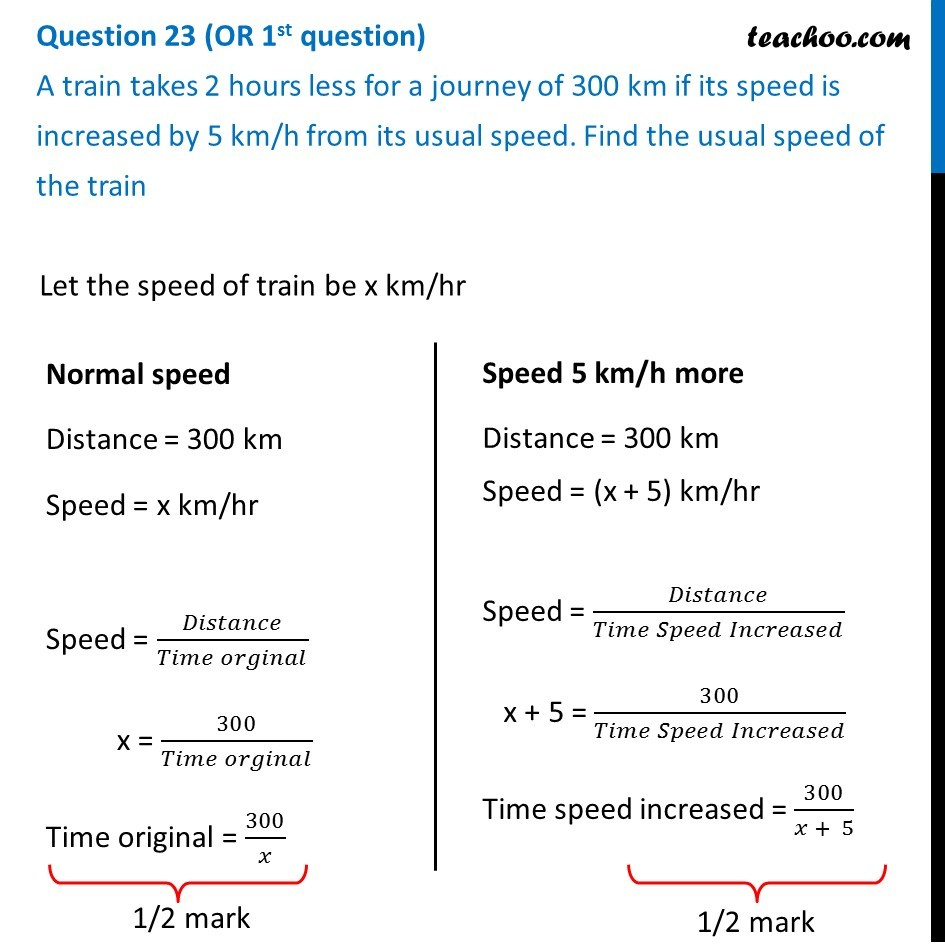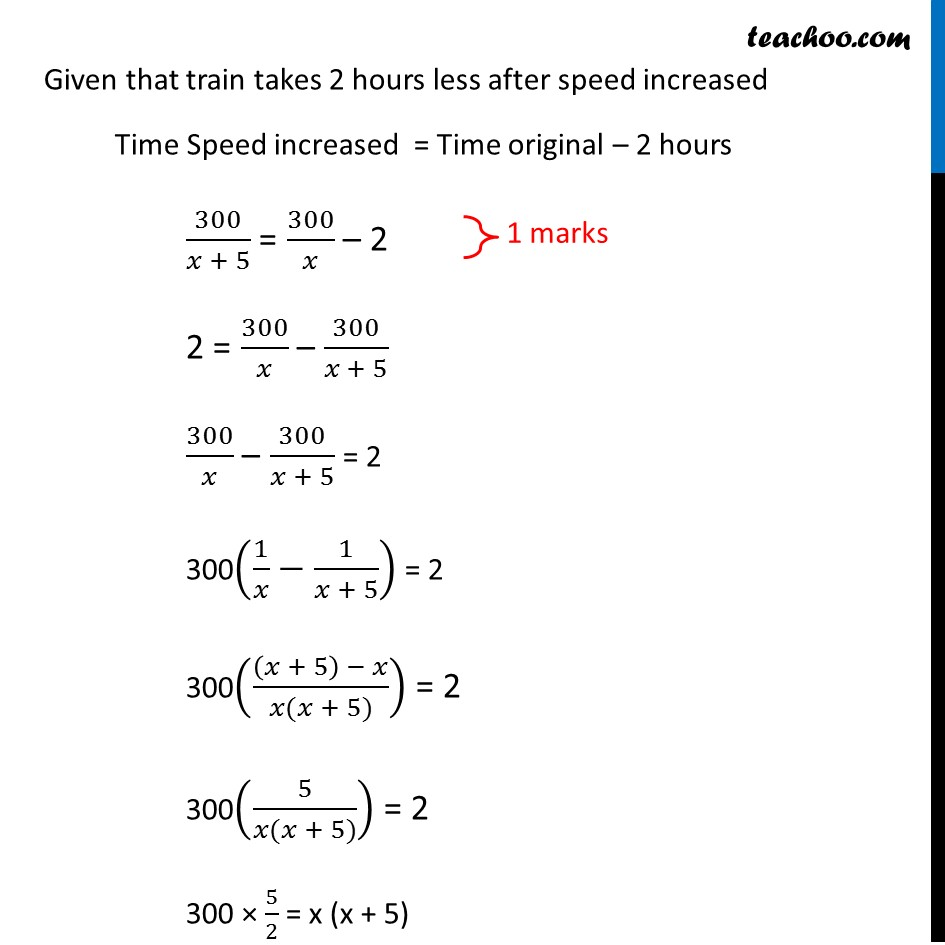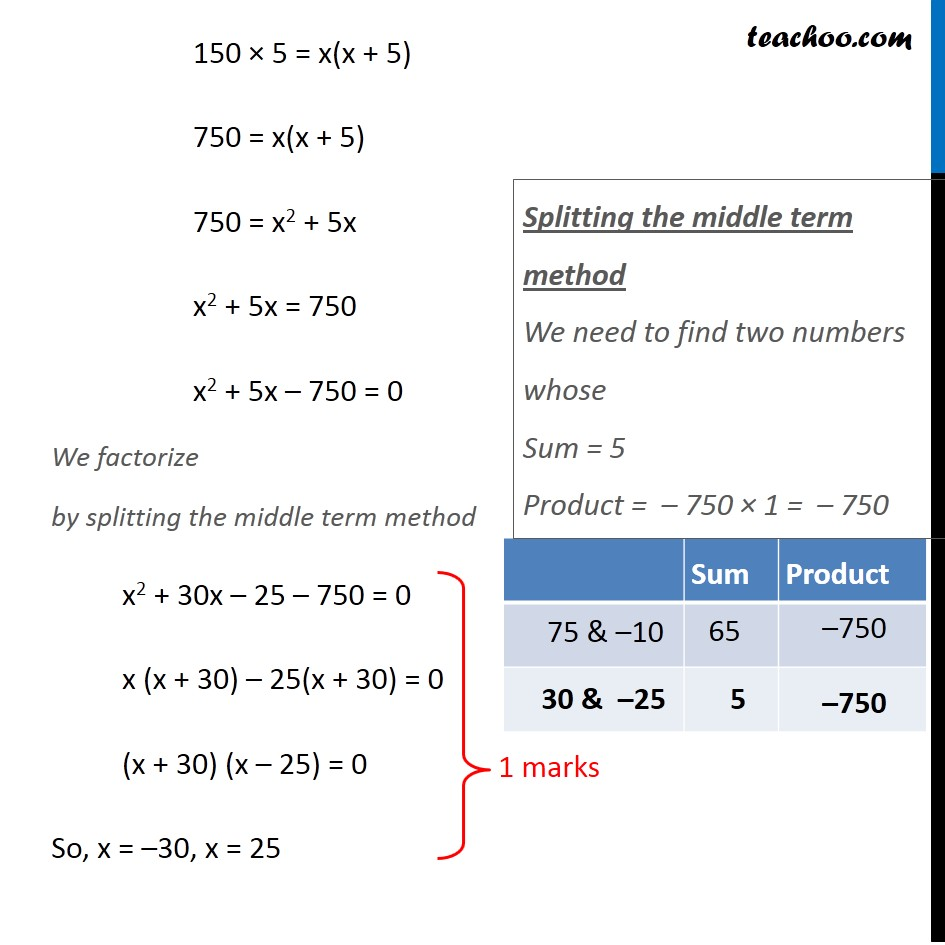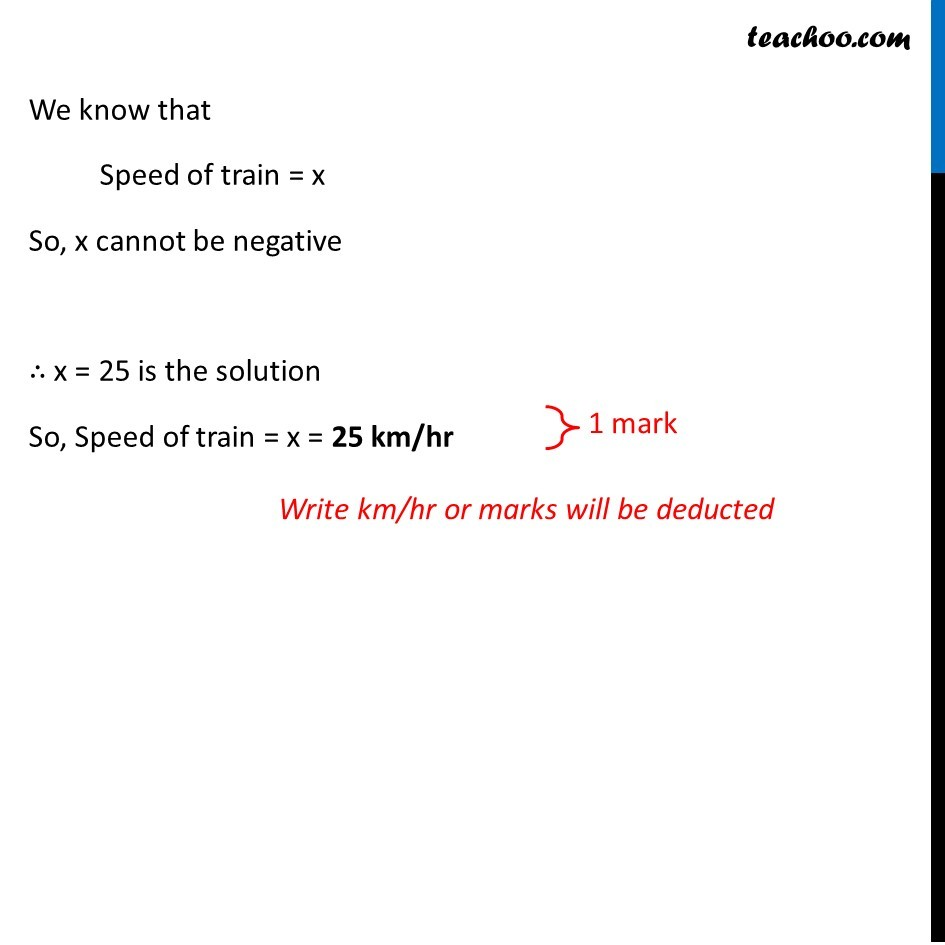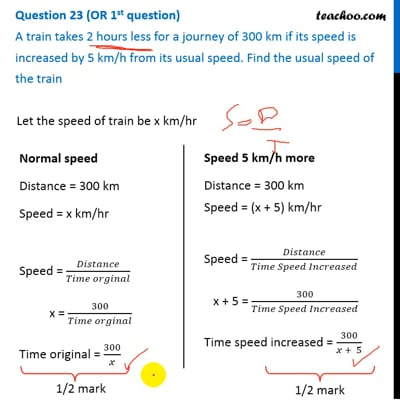This video is only available for Teachoo black users

### Transcript

Question 23 (OR 1st question) A train takes 2 hours less for a journey of 300 km if its speed is increased by 5 km/h from its usual speed. Find the usual speed of the train Let the speed of train be x km/hr Normal speed Distance = 300 km Speed = x km/hr Speed = 𝐷𝑖𝑠𝑡𝑎𝑛𝑐𝑒/(𝑇𝑖𝑚𝑒 𝑜𝑟𝑔𝑖𝑛𝑎𝑙) x = 300/(𝑇𝑖𝑚𝑒 𝑜𝑟𝑔𝑖𝑛𝑎𝑙) Time original = 300/𝑥 Speed 5 km/h more Distance = 300 km Speed = (x + 5) km/hr Speed = 𝐷𝑖𝑠𝑡𝑎𝑛𝑐𝑒/(𝑇𝑖𝑚𝑒 𝑆𝑝𝑒𝑒𝑑 𝐼𝑛𝑐𝑟𝑒𝑎𝑠𝑒𝑑) x + 5 = 300/(𝑇𝑖𝑚𝑒 𝑆𝑝𝑒𝑒𝑑 𝐼𝑛𝑐𝑟𝑒𝑎𝑠𝑒𝑑) Time speed increased = 300/(𝑥 + 5) Given that train takes 2 hours less after speed increased Time Speed increased = Time original – 2 hours 300/(𝑥 + 5) = 300/𝑥 – 2 2 = 300/𝑥 – 300/(𝑥 + 5) 300/𝑥 – 300/(𝑥 + 5) = 2 300(1/𝑥−1/(𝑥 + 5)) = 2 300(((𝑥 + 5) − 𝑥)/(𝑥(𝑥 + 5))) = 2 300(5/(𝑥(𝑥 + 5))) = 2 300 × 5/2 = x (x + 5) 150 × 5 = x(x + 5) 750 = x(x + 5) 750 = x2 + 5x x2 + 5x = 750 x2 + 5x – 750 = 0 We factorize by splitting the middle term method x2 + 30x – 25 – 750 = 0 x (x + 30) – 25(x + 30) = 0 (x + 30) (x – 25) = 0 So, x = –30, x = 25 Splitting the middle term method We need to find two numbers whose Sum = 5 Product = – 750 × 1 = – 750 We know that Speed of train = x So, x cannot be negative ∴ x = 25 is the solution So, Speed of train = x = 25 km/hr We know that Speed of train = x So, x cannot be negative ∴ x = 25 is the solution So, Speed of train = x = 25 km/hr